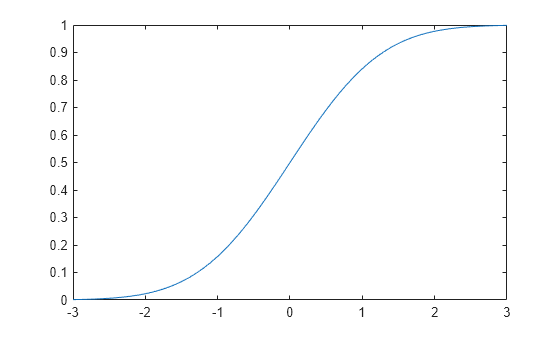## 正态分布

### 概述

Statistics and Machine Learning Toolbox™ 提供了几种处理正态分布的方法。

### 参数

mu (μ)均值$-\infty <\mu <\infty$
sigma (σ)标准差$\sigma \ge 0$

#### 参数估计

$\overline{x}=\sum _{i=1}^{n}\frac{{x}_{i}}{n}$

${s}_{\text{MLE}}^{2}=\frac{1}{n}\sum _{i=1}^{n}{\left({x}_{i}-\overline{x}\right)}^{2}.$

$\overline{x}$ 是样本 x1, x2, …, xn 的样本均值。样本均值是参数 μ 的无偏估计量。但是，s2MLE 是参数 σ2 的有偏估计量，这意味着其预期值不等于参数。

${s}^{2}=\frac{1}{n-1}\sum _{i=1}^{n}{\left({x}_{i}-\overline{x}\right)}^{2}$

• 对于未删失数据，normfitfitdist 计算无偏估计值，mle 计算最大似然估计值。

• 对于删失数据，normfitfitdistmle 计算最大似然估计值。

### 概率密度函数

$y=f\left(x|\mu ,\sigma \right)=\frac{1}{\sigma \sqrt{2\pi }}{e}^{\frac{-{\left(x-\mu \right)}^{2}}{2{\sigma }^{2}}},\text{ }\text{for}\text{\hspace{0.17em}}x\in ℝ.$

### 累积分布函数

$p=F\left(x|\mu ,\sigma \right)=\frac{1}{\sigma \sqrt{2\pi }}{\int }_{-\infty }^{x}{e}^{\frac{-{\left(t-\mu \right)}^{2}}{2{\sigma }^{2}}}dt,\text{ }\text{for}\text{\hspace{0.17em}}x\in ℝ.$

p 是参数为 μσ 的正态分布中的一个观测值落入 (-∞,x] 区间的概率。

$\Phi \left(x\right)=\frac{1}{2}\left(1-\text{erf}\left(-\frac{x}{\sqrt{2}}\right)\right)$

$\text{erf}\left(x\right)=\frac{2}{\sqrt{\pi }}{\int }_{0}^{x}e{}^{-{t}^{2}}dt=2\Phi \left(\sqrt{2}x\right)-1.$

### 示例

#### 拟合正态分布对象

load examgrades x = grades(:,1);

pd = fitdist(x,'Normal')
pd = NormalDistribution Normal distribution mu = 75.0083 [73.4321, 76.5846] sigma = 8.7202 [7.7391, 9.98843] 

#### 计算并绘制正态分布 pdf

x = [-3:.1:3]; y = normpdf(x,0,1);

plot(x,y)#### 绘制标准正态分布 cdf

pd = makedist('Normal')
pd = NormalDistribution Normal distribution mu = 0 sigma = 1 

x = -3:.1:3; p = cdf(pd,x);

plot(x,p)#### 比较 gamma 和正态分布 pdf

gamma 分布具有形状参数 $\mathit{a}$ 和尺度参数 $\mathit{b}$。如果 $\mathit{a}$ 的值较大，gamma 分布非常接近均值 $\mu =\mathit{ab}$、方差 ${\sigma }^{2}=\mathit{a}{\mathit{b}}^{2}$ 的正态分布。

a = 100; b = 5; x = 250:750; y_gam = gampdf(x,a,b);

mu = a*b
mu = 500 
sigma = sqrt(a*b^2)
sigma = 50 
y_norm = normpdf(x,mu,sigma);

plot(x,y_gam,'-',x,y_norm,'-.') title('Gamma and Normal pdfs') xlabel('Observation') ylabel('Probability Density') legend('Gamma Distribution','Normal Distribution')#### 正态分布和对数正态分布之间的关系

pd = makedist('Lognormal','mu',5,'sigma',2)
pd = LognormalDistribution Lognormal distribution mu = 5 sigma = 2 

mean(pd)
ans = 1.0966e+03 

rng('default'); % For reproducibility x = random(pd,10000,1); logx = log(x);

m = mean(logx)
m = 5.0033 

x 的对数的均值接近 xmu 参数，因为 x 具有对数正态分布。

histfit(logx)histfit 使用 fitdist 对数据进行分布拟合。使用 fitdist 获得在拟合中使用的参数。

pd_normal = fitdist(logx,'Normal')
pd_normal = NormalDistribution Normal distribution mu = 5.00332 [4.96445, 5.04219] sigma = 1.98296 [1.95585, 2.01083] 

#### 比较 Student t 和正态分布 pdf

Student t 分布是依赖于单参数 ν（自由度）的曲线族。随着自由度 ν 趋向无穷，t 分布逼近标准正态分布。

x = [-5:0.1:5]; y1 = tpdf(x,5); y2 = tpdf(x,15);

z = normpdf(x,0,1);

plot(x,y1,'-.',x,y2,'--',x,z,'-') legend('Student''s t Distribution with \nu=5', ... 'Student''s t Distribution with \nu=15', ... 'Standard Normal Distribution','Location','best') xlabel('Observation') ylabel('Probability Density') title('Student''s t and Standard Normal pdfs')### 相关分布

• Binomial Distribution - 二项分布对 n 次重复试验的成功总数和成功概率 p 进行建模。随着 n 的增长，二项分布可以用 µ = npσ2 = np(1–p) 的正态分布来逼近。请参阅Compare Binomial and Normal Distribution pdfs

• Birnbaum-Saunders Distribution - 如果 x 具有参数为 β 和 γ 的 Birnbaum-Saunders 分布，则

$\frac{\left(\sqrt{x}{\beta }}-\sqrt{\beta }{x}}\right)}{\gamma }$

具有标准正态分布。

• Chi-Square Distribution - 卡方分布是平方和、独立、标准正态随机变量的分布。如果一组（包含 n 个）观测值呈正态分布，方差为 σ2、样本方差为 s2，则 (n–1)s22 具有自由度为 n–1 的卡方分布。normfit 函数使用此关系来计算正态参数 σ2 的估计的置信区间。

• Extreme Value Distribution - 极值分布适用于对尾部呈指数急剧衰减的分布（如正态分布）中的最小值或最大值建模。

• Gamma Distribution - gamma 分布具有形状参数 a 和尺度参数 b。如果 a 的值较大，gamma 分布非常接近均值 μ = ab、方差 σ2 = ab2 的正态分布。gamma 分布仅对正实数才有密度。请参阅比较 gamma 和正态分布 pdf

• Half-Normal Distribution - 半正态分布是折叠正态分布和截断正态分布的特例。如果随机变量 Z 具有标准正态分布，则 $X=\mu +\sigma |Z|$ 为具有参数 μ 和 σ 的半正态分布。

• Logistic Distribution - 逻辑分布用于增长模型和逻辑回归。与正态分布相比，逻辑分布的尾部更长、峰度更高。

• Lognormal Distribution - 如果 X 遵循具有参数 µ 和 σ 的对数正态分布，则 log(X) 遵循具有均值 µ 和标准差 σ 的正态分布。请参阅正态分布和对数正态分布之间的关系

• Multivariate Normal Distribution - 多元正态分布是一元正态分布的双变量或多变量泛化。它是相关变量的随机向量的分布，其中每个元素都呈一元正态分布。在最简单的情形下，各变量之间没有相关性，向量的元素是独立的一元正态随机变量。

• 泊松分布 - 泊松分布是接受非负整数值的单参数离散分布。参数 λ 既是分布的均值，也是分布的方差。随着 λ 的增大，泊松分布可以用 µ = λσ2 = λ 的正态分布来逼近。

• Rayleigh Distribution - 瑞利分布是 Weibull 分布的特例，应用于通信理论中。如果粒子在 xy 方向上的分量速度是两个独立的正态随机变量（均值为零并具有方差齐性），则粒子在每单位时间行进的距离遵循瑞利分布。

• Stable Distribution - 正态分布是稳定分布的特例。第一个形状参数 α = 2 的稳定分布对应于正态分布。

$N\left(\mu ,{\sigma }^{2}\right)=S\left(2,0,\frac{\sigma }{\sqrt{2}},\mu \right)\text{\hspace{0.17em}}.$

• Student's t Distribution - Student t 分布是一个依赖单参数 ν（自由度）的曲线族。随着自由度 ν 趋向无穷，t 分布逼近标准正态分布。请参阅比较 Student t 和正态分布 pdf

如果 x 是大小为 n 的随机样本，来自均值为 μ 的正态分布，则统计量

$t=\frac{\overline{x}-\mu }{s/\sqrt{n}}$

（其中 $\overline{x}$ 是样本均值，s 是样本标准差）具有包含 n–1 个自由度的 Student t 分布。

• t Location-Scale Distribution - t 位置尺度分布适用于对尾部比正态分布重（更容易出现离群值）的数据分布进行建模。当形状参数 ν 趋向无穷时，它逼近正态分布。

 Abramowitz, M., and I. A. Stegun. Handbook of Mathematical Functions. New York: Dover, 1964.

 Evans, M., N. Hastings, and B. Peacock. Statistical Distributions. 2nd ed. Hoboken, NJ: John Wiley & Sons, Inc., 1993.

 Lawless, J. F. Statistical Models and Methods for Lifetime Data. Hoboken, NJ: Wiley-Interscience, 1982.

 Marsaglia, G., and W. W. Tsang. “A Fast, Easily Implemented Method for Sampling from Decreasing or Symmetric Unimodal Density Functions.” SIAM Journal on Scientific and Statistical Computing. Vol. 5, Number 2, 1984, pp. 349–359.

 Meeker, W. Q., and L. A. Escobar. Statistical Methods for Reliability Data. Hoboken, NJ: John Wiley & Sons, Inc., 1998.